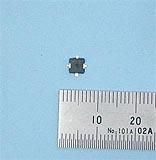コスモウェーブ## 高周波増幅用ＭｏｓＦＥＴ　NE5510279A

NE5510279A

1,400円(税込1,512円)

NE5510279A

DESCRIPTION
The NE5510279A is an N-channel silicon power MOS FET specially designed as the transmission power amplifier for 4.8 V GSM 1 800 handsets. Dies are manufactured using our NEWMOS technology (our 0.6 μm WSi gate laterally diffused MOS FET) and housed in a surface mount package. The device can deliver 33.0 dBm output power with 47% power added efficiency at 1.8 GHz under the 4.8 V supply voltage.

FEATURES
• High output power : Pout = 35.5 dBm TYP. (VDS = 4.8 V,
IDset = 300 mA, f = 900 MHz, Pin = 25 dBm)
: Pout = 33.0 dBm TYP. (VDS = 4.8 V, IDset = 300 mA, f = 1.8 GHz, Pin = 25 dBm)
• High power added efficiency : ηadd = 65% TYP. (VDS = 4.8 V, IDset = 300 mA, f = 900 MHz, Pin = 25 dBm) : ηadd = 47% TYP. (VDS = 4.8 V, IDset = 300 mA, f = 1.8 GHz, Pin = 25 dBm)
• High linear gain : GL = 16.0 dB TYP. (VDS = 4.8 V, IDset = 300 mA, f = 900 MHz, Pin = 10 dBm) : GL = 10.0 dB TYP. (VDS = 4.8 V, IDset = 300 mA, f = 1.8 GHz, Pin = 10 dBm)
• Surface mount package : 5.7 × 5.7 × 1.1 mm MAX.
• Single supply : VDS = 3.0 to 8.0 V

APPLICATIONS
• Digital cellular phones : 4.8 V GSM 1 800 class 1 handsets
• Others : General purpose amplifiers for 1.6 to 2.0 GHz TDMA applications

イメージはありません。

レビューはありません。